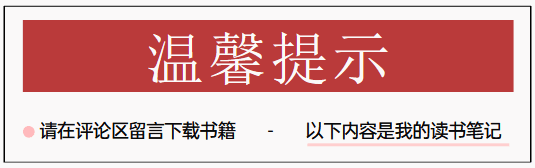1.2.2　C语言编程原理{

m_R=r;

}

//根据一个矩形创建圆

CFigCircle::CFigCircle(int x1,int y1,int x2,int y2):CFigEllipse(x1,y1,x2,y2)

{

m_X1=(x2+x1)/2;

m_Y1=(y2+y1)/2;

m_R=((x2-x1)+(y2-y1))/2;

m_R=m_R＞0?m_R:-m_R;

}

void CFigCircle::Draw(CDC *pDC)